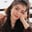Related Tags

pandas

# How to draw a lag plot in pandasFouzia Bashir

## Overview

A special kind of scatter plot is used to draw two variables X & Y with lag. One set of observations X is plotted in time series parallel to the second Y.

### The pandas.plotting.lag_plot() function

The lag_plot() function is used in pandas to draw a lag plot. It takes a time series, a lag, a matplotlib axis object, and some additional keyword argument values. This method returns a matplotlib.axis.Axes instance.

### Syntax

pandas.plotting.lag_plot(series, lag=1, ax=None, **kwds)

### Parameters

It takes the following argument values.

• series: This is an instance of a time series.
• lag: This is an integer value, Default=1, which shows a lag between every point of the scatter plot.
• ax: This is a matplotlib axis object, Default=None.
• **kwds: These are the additional arguments for the scatter plot.

### Return value

It returns an instance of the matplotlib.axis.Axes module.

### Explanation

In this code snippet, we create use the pandas.plotting.lag_plot() function to create a lag plot.

# import python libraries in program
import pandas as pd
import numpy as np
import matplotlib.pyplot as plt
# create a ndarray of cummulative sum of numbers
x = np.cumsum(np.random.normal(loc=1, scale=5, size=25))
# create a series of above created random values
s = pd.Series(x)
# invoke lag_plot() function to draw a scaller plot
pd.plotting.lag_plot(s, lag=3)
# save output image as png
plt.savefig("output/lagplot.png")
• Line 2–4: We import pandas, NumPy, and matplotlib libraries in the program.
• Line 6: The np.cumsum() function will generate a cumulative sum of random values.
• Line 8: We use the pd.Series(x) function to create a series of the above-created cumulative values.
• Line 10: We create a scatter plot with s as series and lag=3.
• Line 12: We save the graph output as a PNG file in the output folder.

RELATED TAGS

pandas

CONTRIBUTORFouzia Bashir
RELATED COURSES

View all Courses

Keep Exploring

Learn in-demand tech skills in half the time